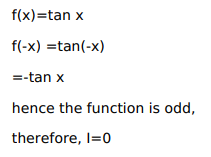# Mark against the correct answer in the following:

Question:

Mark $(\sqrt{)}$ against the correct answer in the following:

$\int_{-\pi}^{\pi} \tan x d x=?$

A. 2

B. $\frac{1}{2}$

C. $-2$

D. 0

Solution: